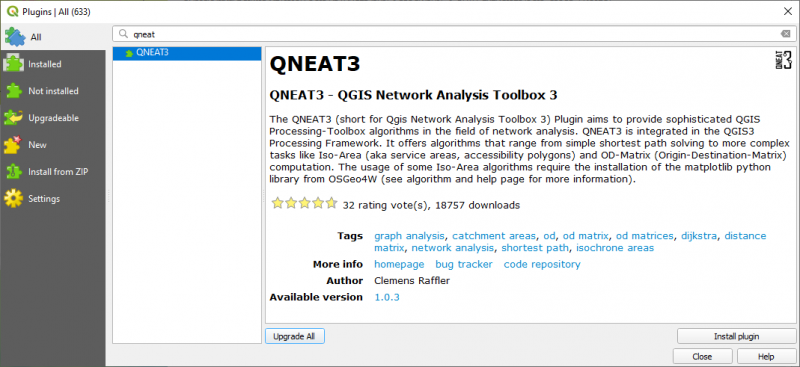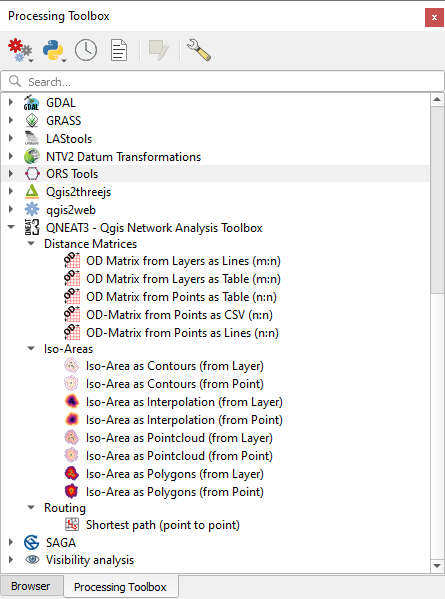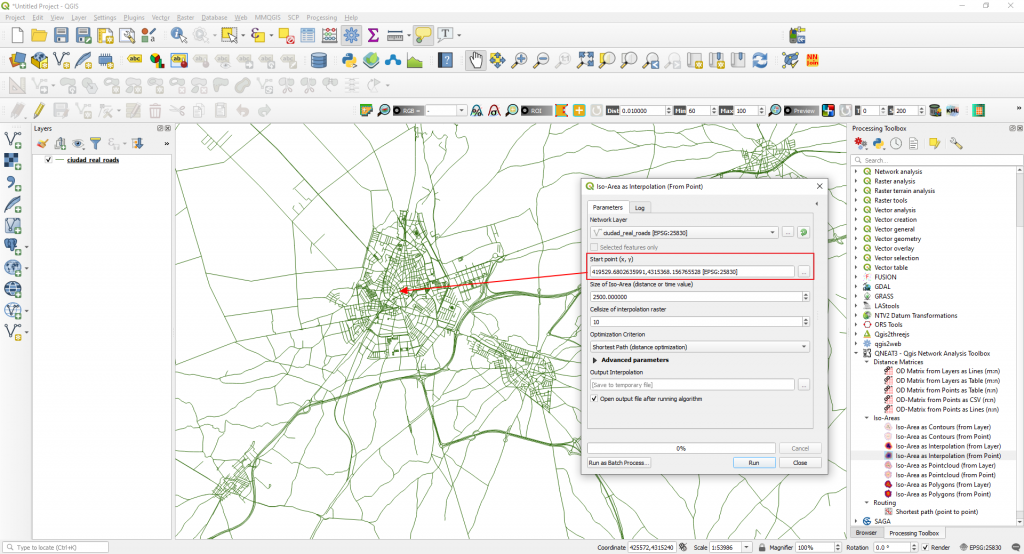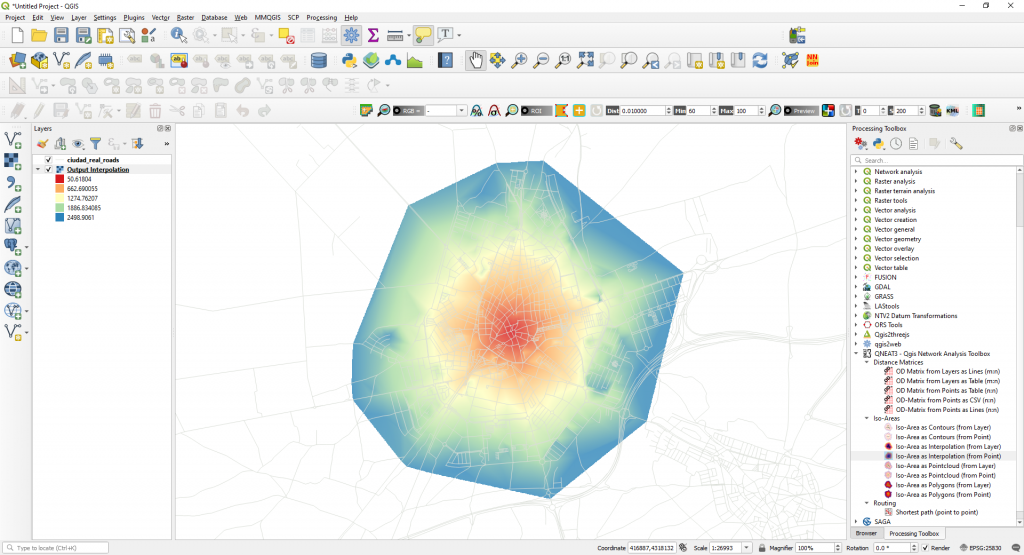QNEAT3 is a QGIS plugin integrated in the QGIS3 Processing Framework. It offers advanced network analysis algorithms that range from simple shortest path solving to more complex tasks like Isochrone Area (aka service areas, accessibility polygons) and OD-Matrix (Origin-Destination-Matrix)computation.

The author of this plugin is Clemens Raffler (Mobility Researcher, GIS-Enthusiast Vienna, Austria). For more information you can check the next link: https://root676.github.io/

You can install this plugin using the QGIS Plugin Manager.After the plugin is installed, go to the Processing Toolbox and check for the algorithms.The algorithms:

1.  Shortest Path (Point to Point) – (https://root676.github.io/ShortestPathAlgs.html)

This is the standard Processing Network analysis algorithm. This algorithm implements the Dijkstra-Search to return the shortest path between two points on a given network dataset.
It accounts for points outside of the network (eg. non-network-elements) and calculates separate entry and exit costs.

2. Iso-Area (as Contours, as Interpolation, as PointCloud, as Polygons) – (https://root676.github.io/IsoAreaAlgs.html)

Iso-Area as Interpolation (from Point) – example

This algorithm implements iso-area analysis to return the network-distance interpolation for a maximum cost level on a given network dataset for a manually chosen point.

You need to select the following parameters in the algorithm dialog window.

Startpoint
Maximum cost level for Iso-Area
Cellsize in Meters (increase default when analyzing larger networks)
Cost Strategy (distance or time) in the Optimization Criterion.We just created a service area around a start point taking into consideration a maximum distance of 2500 m. Now we can locate in our network the areas covered within a 2.5 km walking distance. This is because in the Advanced Parameters of the algorithm dialog box a speed of 5 km/h was set.3. Distance Matrices – (https://root676.github.io/OdMatrixAlgs.html)

This algorithm compute the travel cost on network (either routed distance or routed time) between all combination of points in a layer.

Source of this post: QNEAT3 QGIS Network Analysis Toolbox 3 – https://root676.github.io/

Quality training taught by professionals